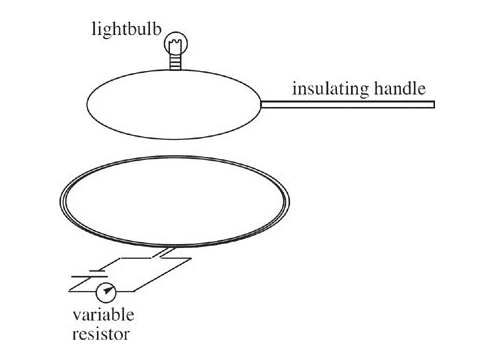# Why wouldn't rotating the handle 90 degrees induce an EMF?

## Homework StatementThere is some current in the bottom coil.
The question asks which would not be able to light the lightbulb
- rotating the insulating handle 90 degrees
- increasing resistance of variable resistor
-reducing resistance of variable resistor
- moving the insulating handle towards and away from the current carrying coil.

## Homework Equations

emf = change in magnetic flux over time.
right hand rule for current carrying wire
B = (permeability of free space * current)/ (2pi * radius)
magnetic flux = BA

## The Attempt at a Solution

Rotating the handle 90 degrees would change the area exposed to the magnetic field. All the other choices would change the strength of the magnetic field. Wouldn't all of these choices be able to light the lightbulb?

#### Attachments

Doc Al
Mentor
Rotating the handle 90 degrees would change the area exposed to the magnetic field.
Perhaps they meant rotating the handle about a "vertical" axis, keeping the loop in the same plane.

Chandra Prayaga